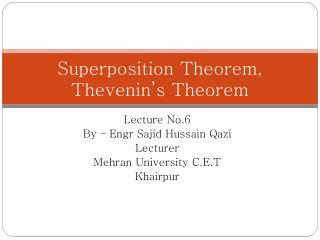DownloadDownload PresentationSuperposition Theorem, Thevenin’s Theorem

# Superposition Theorem, Thevenin’s Theorem

Télécharger la présentation## Superposition Theorem, Thevenin’s Theorem

- - - - - - - - - - - - - - - - - - - - - - - - - - - E N D - - - - - - - - - - - - - - - - - - - - - - - - - - -
##### Presentation Transcript

1. Superposition Theorem,Thevenin’s Theorem Lecture No.6 By – EngrSajidHussainQazi Lecturer Mehran University C.E.T Khairpur

2. Superposition Theorem • The superposition theorem, like the methods of the last lecture , can be used to find the solution to networks with two or more sources that are not in series or parallel. • The most obvious advantage of this method is that it does not require the use of a mathematical technique such as determinants to find the required voltages or currents. • Instead, each source is treated independently, and the algebraic sum is found to determine a particular unknown quantity of the network. The superposition theorem states the following: “The current through, or voltage across an element in a linear bilateral network is equal to the algebraic sum of the currents or voltages produced independently by each source.” • To consider the effects of each source independently requires that sources be removed and replaced without affecting the final result. • To remove a voltage source when applying this theorem; voltage source must be set to zero (or short circuited) • Removing a current source requires that its terminals be opened (open circuit). Engr. S.H.Qazi

3. The total current through any portion of the network is equal to the algebraic sum of the currents produced independently by each source. • That is, for a two-source network, if the current produced by one source is in one direction, while that produced by the other is in the opposite direction through the same resistor, the resulting current is the difference of the two currents. • If the individual currents are in the same direction, the resulting current is the sum of two currents. Engr. S.H.Qazi

4. EXAMPLE - Determine I1 for the network of Fig. Setting E=0 or short circuit As shown in Fig, the source current will choose the short circuit path, and hence I′1 = 0 A. Setting I to zero amperes will result in the network of Fig-a, with the current source replaced by an open circuit. Applying Ohm’s law, Engr. S.H.Qazi

5. Since I′1 and I″1 have the same defined direction in Fig. above, the current I1 is the sum of the two, and EXAMPLE - Using superposition, determine the current through the 4- resistor of Fig. Solution: Considering the effects of a 54-V source Engr. S.H.Qazi

6. Now, Considering the effects of a 48-V source Since I′3 and I″3 have opposite defined direction in Fig. above, the current I3 is the difference of the two, and Engr. S.H.Qazi

7. EXAMPLE Using the principle of superposition, find the current I2 through the 12-k resistor of Fig Solution: Considering the effect of the 6-mA current source Using current divider rule, Considering the effect of the 9-volt battery source, Since I′2 and I″2 have the same defined direction in Fig. above, the current I1 is the sum of the two, and Engr. S.H.Qazi

8. EXAMPLE Using superposition, find the current through the 6-ohm resistor of the network of Fig Engr. S.H.Qazi

9. Thevenin’s Theorem Thevenin’s theorem states the following: “Any two-terminal, linear bilateral dc network can be replaced by an equivalent circuit consisting of a voltage source and a series resistor.” as shown in Fig Engr. S.H.Qazi

10. In Fig. 1(a), for example, the network within the container has only two terminals available to the outside world, labeled a and b. • It is possible using Thevenin’s theorem to replace everything in the container with one source and one resistor, as shown in Fig. 1(b), and maintain the same terminal characteristics at terminals a and b. • The load will receive the same current, voltage, and power from either configuration of Fig. 1. Figure-1 Engr. S.H.Qazi

11. Before we examine the steps involved in applying this theorem, it is important to ensure that the entire network, except RL, is to be replaced by a single series resistor and battery as shown in Fig. 1(b). • The values of these two elements of the Thevenin equivalent circuit must be chosen to ensure that the resistor RL will react to the network of Fig. 1(a) in the same manner as to the network of Fig. 2(b). • In other words, the current through or voltage across RL must be the same for either network for any value of RL. The following sequence of steps will lead to the application of Thevenin’s Theorem Remove the load resistor RL temporarily from the network where Thevenin’s theorem is to be used. Mark the terminals of the remaining two-terminal network. Engr. S.H.Qazi

12. 3. Calculate RTh by first setting all sources to zero (voltage sources are replaced by short circuits, and current sources by open circuits) and then finding the resultant resistance between the two marked terminals. 4. Calculate ETh by first returning all sources to their original position and finding the open-circuit voltage between the marked terminals. 5. Draw the Thevenin equivalent circuit with the portion of the circuit previously removed replaced between the terminals of the equivalent circuit. This step is indicated by the placement of the resistor RL between the open terminals. Engr. S.H.Qazi